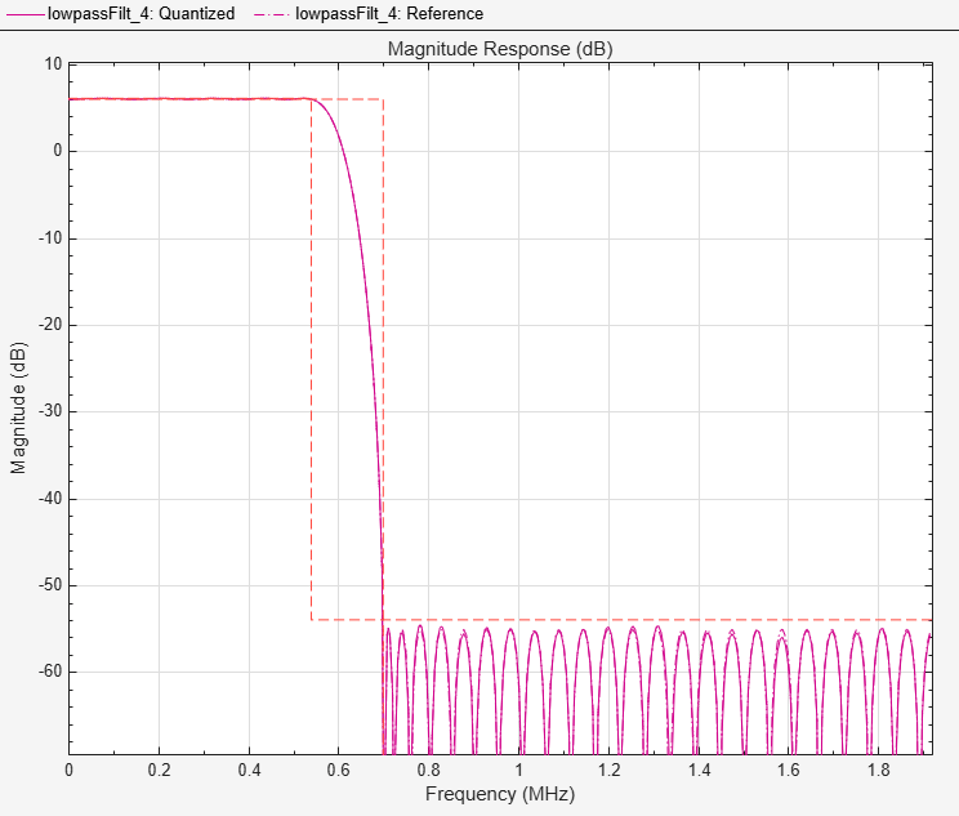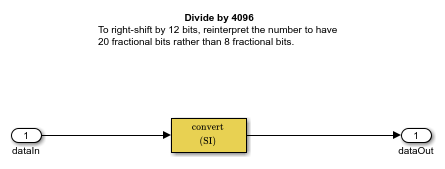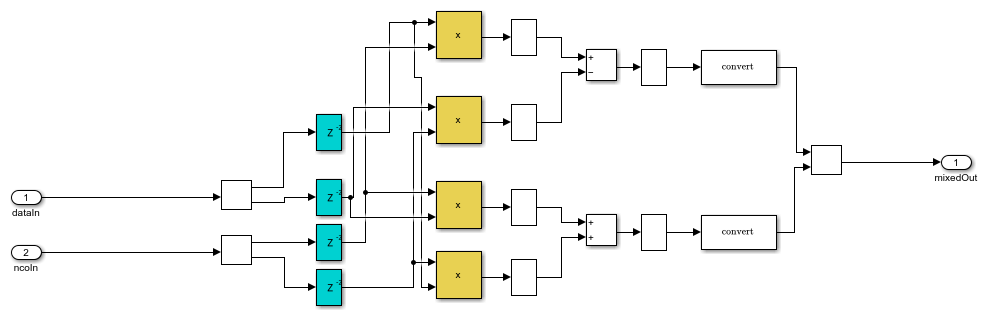# HDL Implementation of a Digital Up-Converter for LTE

This example shows how to design a digital up-converter (DUC) for radio communication applications such as LTE, and generate HDL code.

### Introduction

DUCs are widely used in digital communication transmitters to convert baseband signals to radio frequency (RF) or intermediate frequency (IF) signals. The DUC operation increases the sample rate of the signal and shifts it to a higher frequency to facilitate subsequent processing stages. The DUC in this example performs sample rate conversion using a four-stage filter chain followed by complex frequency translation. The example starts by designing the DUC with DSP System Toolbox™ functions in floating point. Then, each stage is converted to fixed point, and used in a Simulink® model that generates synthesizable HDL code. The example uses these two test signals to demonstrate and verify the DUC operation:

• A sinusoid that is modulated onto a 32 MHz IF carrier.

• An LTE downlink signal with a bandwidth of 1.4 MHz, modulated onto a 32 MHz IF carrier.

The example downconverts the outputs of the floating-point and fixed-point DUCs, and compares the signal quality of the two outputs.

Finally, the example presents implementation of the filter chain for FPGAs, and synthesis results.

This example uses `DUCTestUtils`, a helper class that contains functions for generating stimulus and analyzing the DUC output. For more information, see the `DUCTestUtils.m` file.

### DUC Structure

The DUC consists of an interpolating filter chain, numerically controlled oscillator (NCO), and mixer. The filter chain consists of a lowpass interpolator, halfband interpolator, CIC compensation interpolator (FIR), CIC interpolator, and CIC gain correction.

The overall response of the filter chain is equivalent to that of a single interpolation filter with the same specification. However, splitting the filter into multiple interpolation stages results in a more efficient design that uses fewer hardware resources.

The first lowpass interpolator implements the precise `Fpass` and `Fstop` characteristics of the DUC. The halfband filter is an intermediate interpolator. The lower sampling rates at the beginning of the chain mean the earlier filters can optimize resource use by sharing multipliers. The CIC compensation interpolator improves the spectral response by compensating for the later CIC droop while interpolating by two. The CIC interpolator provides a large interpolation factor, which meets the filter chain upsampling requirements.

This figure shows a block diagram of the DUC.The sample rate of the input to the DUC is 1.92 Msps, and the output sample rate is 122.88 Msps. These rates give an overall interpolation factor of 64. LTE receivers use 1.92 Msps as the typical sampling rate for cell search and master information block (MIB) recovery. The DUC filters are designed to suit this application. The DUC is optimized to run at a clock rate of 122.88 MHz.

### DUC Design

This section explains how to design the DUC using floating-point operations and filter-design functions in MATLAB®. The DUC object enables you to specify several characteristics that define the response of the cascade for the four filters, including passband and stopband frequencies, passband ripple, and stopband attenuation.

DUC Parameters

This example designs the DUC filter characteristics to meet these desired response values for the given input sampling rate and carrier frequency.

```FsIn = 1.92e6; % Sampling rate at input to DUC Fc = 32e6; % Carrier frequency Fpass = 540e3; % Passband frequency, equivalent to 36*15kHz LTE subcarriers Fstop = 700e3; % Stopband frequency Ap = 0.1; % Passband ripple Ast = 60; % Stopband attenuation ```

First Lowpass Interpolator

This filter interpolates by two, and operates at the lowest sampling rate of the filter chain. The low sample rate means this filter can use resource sharing for an efficient hardware implementation.

```lowpassParams.FsIn = FsIn; lowpassParams.InterpolationFactor = 2; lowpassParams.FsOut = FsIn*lowpassParams.InterpolationFactor; lowpassSpec = fdesign.interpolator(lowpassParams.InterpolationFactor, ... 'lowpass','Fp,Fst,Ap,Ast',Fpass,Fstop,Ap,Ast,lowpassParams.FsOut); lowpassFilt = design(lowpassSpec,'SystemObject',true) ```
```lowpassFilt = dsp.FIRInterpolator with properties: InterpolationFactor: 2 NumeratorSource: 'Property' Numerator: [0.0020 0.0021 4.9115e-04 -0.0027 -0.0050 ... ] Use get to show all properties ```

Display the magnitude response of the lowpass filter without gain correction.

```ducPlots.lowpass = fvtool(lowpassFilt,'Fs',FsIn*2,'Legend','off'); DUCTestUtils.setPlotNameAndTitle('Lowpass Interpolator'); ```Second Halfband Interpolator

The halfband filter provides efficient interpolation by two. Halfband filters are efficient for hardware because approximately half of their coefficients are equal to zero, and those multipliers are excluded from the hardware implementation.

``` hbParams.FsIn = lowpassParams.FsOut; hbParams.InterpolationFactor = 2; hbParams.FsOut = lowpassParams.FsOut*hbParams.InterpolationFactor; hbParams.TransitionWidth = hbParams.FsIn - 2*Fstop; hbParams.StopbandAttenuation = Ast; hbSpec = fdesign.interpolator(hbParams.InterpolationFactor,'halfband', ... 'TW,Ast', ... hbParams.TransitionWidth, ... hbParams.StopbandAttenuation, ... hbParams.FsOut); hbFilt = design(hbSpec,'SystemObject',true) ```
```hbFilt = dsp.FIRInterpolator with properties: InterpolationFactor: 2 NumeratorSource: 'Property' Numerator: [0.0178 0 -0.1129 0 0.5953 1 0.5953 0 -0.1129 0 ... ] Use get to show all properties ```

Visualize the magnitude response of the halfband interpolation.

```ducFilterChain = dsp.FilterCascade(lowpassFilt,hbFilt); ducPlots.hbFilt = fvtool(lowpassFilt,hbFilt,ducFilterChain, ... 'Fs',[FsIn*2,FsIn*4,FsIn*4]); legend( ... 'Lowpass interpolator', ... 'Halfband interpolator', ... 'Lowpass and halfband'); DUCTestUtils.setPlotNameAndTitle('Halfband Interpolator'); ```CIC Compensation Interpolator

Because the magnitude response of the last CIC filter has a significant droop within the passband region, the example uses an FIR-based droop compensation filter to flatten the passband response. The droop compensator has the same properties as the CIC interpolator. This filter implements interpolation by a factor of two, so you must also specify bandlimiting characteristics for the filter. Also, specify the CIC interpolator properties that are used for this compensation filter as well as the later CIC interpolator.

Use the `design` function to return a filter System object with the specified characteristics.

```compParams.FsIn = hbParams.FsOut; compParams.InterpolationFactor = 2; % CIC compensation interpolation factor compParams.FsOut = compParams.FsIn*compParams.InterpolationFactor; % New sampling rate compParams.Fpass = 1/2*compParams.FsIn + Fpass; % CIC compensation passband frequency compParams.Fstop = 1/2*compParams.FsIn + 1/4*compParams.FsIn; % CIC compensation stopband frequency compParams.Ap = Ap; % Same passband ripple as overall filter compParams.Ast = Ast; % Same stopband attenuation as overall filter cicParams.InterpolationFactor = 8; % CIC interpolation factor cicParams.DifferentialDelay = 1; % CIC interpolator differential delay cicParams.NumSections = 3; % CIC interpolator number of integrator and comb sections compSpec = fdesign.interpolator(compParams.InterpolationFactor,'ciccomp', ... cicParams.DifferentialDelay, ... cicParams.NumSections, ... cicParams.InterpolationFactor, ... 'Fp,Fst,Ap,Ast', ... compParams.Fpass,compParams.Fstop,compParams.Ap,compParams.Ast, ... compParams.FsOut); compFilt = design(compSpec,'SystemObject',true) ```
```compFilt = dsp.FIRInterpolator with properties: InterpolationFactor: 2 NumeratorSource: 'Property' Numerator: [-0.0012 0.0078 0.0030 -0.0141 0.0125 0.0118 ... ] Use get to show all properties ```

Plot the response of the CIC compensation interpolator.

```ducFilterChain = dsp.FilterCascade(lowpassFilt,hbFilt,compFilt); ducPlots.cicComp = fvtool(lowpassFilt,hbFilt,compFilt,ducFilterChain, ... 'Fs',[FsIn*2,FsIn*4,FsIn*8,FsIn*8]); legend( ... 'Lowpass interpolator', ... 'Halfband interpolator', ... 'CIC compensation interpolator', ... 'Lowpass, halfband, and CIC compensation'); DUCTestUtils.setPlotNameAndTitle('CIC Compensation Interpolator'); ```CIC Interpolator

The last filter stage is implemented as a CIC interpolator because of this type of filter's ability to efficiently implement a large decimation factor. The response of a CIC filter is similar to a cascade of moving average filters, but a CIC filter uses no multiplication or division. As a result, the CIC filter has a large DC gain.

```cicParams.FsIn = compParams.FsOut; cicParams.FsOut = cicParams.FsIn*cicParams.InterpolationFactor; cicFilt = dsp.CICInterpolator(cicParams.InterpolationFactor, ... cicParams.DifferentialDelay,cicParams.NumSections) %#ok<*NOPTS> ```
```cicFilt = dsp.CICInterpolator with properties: InterpolationFactor: 8 DifferentialDelay: 1 NumSections: 3 FixedPointDataType: 'Full precision' ```

Visualize the magnitude response of the CIC interpolation. CIC filters use fixed-point arithmetic internally, so `fvtool` plots both the quantized and unquantized responses.

```ducFilterChain = dsp.FilterCascade(lowpassFilt,hbFilt,compFilt,cicFilt); ducPlots.cicInter = fvtool(lowpassFilt,hbFilt,compFilt,cicFilt,ducFilterChain, ... 'Fs',[FsIn*2,FsIn*4,FsIn*8,FsIn*64,FsIn*64]); legend( ... 'First halfband interpolator', ... 'Second halfband interpolator', ... 'CIC compensation interpolator', ... 'CIC interpolator: quantized', ... 'CIC interpolator: reference', ... 'Overall response: quantized', ... 'Overall response: reference'); DUCTestUtils.setPlotNameAndTitle('Lowpass, Halfband, CIC Compensation, and CIC Interpolator'); ```Every interpolator has a DC gain that is determined by its interpolation factor. The CIC interpolator has a larger gain than other filters. Call the `gain` function to get the gain factor of this filter.

```% Because the CIC gain is a power of two, a hardware implementation can easily % correct for the gain factor by using a shift operation. % For analysis purposes, the example represents the gain correction by using % a one-tap |dsp.FIRFilter| System object. Combine the filter chain and the % gain correction filter into a |dsp.FilterCascade| System object. cicGain = gain(cicFilt) Gain = lowpassParams.InterpolationFactor* ... hbParams.InterpolationFactor*compParams.InterpolationFactor* ... cicParams.InterpolationFactor*cicGain; GainCorr = dsp.FIRFilter('Numerator',1/Gain) ```
```cicGain = 64 GainCorr = dsp.FIRFilter with properties: Structure: 'Direct form' NumeratorSource: 'Property' Numerator: 2.4414e-04 InitialConditions: 0 Use get to show all properties ```

Plot the overall chain response with and without gain correction.

```ducPlots.overallResponse = fvtool(ducFilterChain,dsp.FilterCascade(ducFilterChain,GainCorr), ... 'Fs',[FsIn*64,FsIn*64]); legend( ... 'No gain correction (quantized)', ... 'No gain correction (reference)', ... 'Gain correction (quantized)', ... 'Gain correction (reference)'); DUCTestUtils.setPlotNameAndTitle('Overall DUC Chain Response'); ```### Fixed-Point Conversion

The frequency response of the floating-point DUC filter chain now meets the specification. Next, quantize each filter stage to use fixed-point types and analyze them to confirm that the filter chain still meets the specification.

Filter Quantization

This example uses 16-bit coefficients, which are sufficient to meet the specification. Using fewer than 18 bits for the coefficients minimizes the number of DSP blocks that are required for an FPGA implementation. The input to the DUC filter chain is 16-bit data with 15 fractional bits. The filter outputs are 18-bit values, which provide extra headroom and precision in the intermediate signals.

```% First Lowpass Interpolator lowpassFilt.FullPrecisionOverride = false; lowpassFilt.CoefficientsDataType = 'Custom'; lowpassFilt.CustomCoefficientsDataType = numerictype([],16,15); lowpassFilt.ProductDataType = 'Full precision'; lowpassFilt.AccumulatorDataType = 'Full precision'; lowpassFilt.OutputDataType = 'Custom'; lowpassFilt.CustomOutputDataType = numerictype([],18,14); % Halfband hbFilt.FullPrecisionOverride = false; hbFilt.CoefficientsDataType = 'Custom'; hbFilt.CustomCoefficientsDataType = numerictype([],16,14); hbFilt.ProductDataType = 'Full precision'; hbFilt.AccumulatorDataType = 'Full precision'; hbFilt.OutputDataType = 'Custom'; hbFilt.CustomOutputDataType = numerictype([],18,14); % CIC Compensation Interpolator compFilt.FullPrecisionOverride = false; compFilt.CoefficientsDataType = 'Custom'; compFilt.CustomCoefficientsDataType = numerictype([],16,14); compFilt.ProductDataType = 'Full precision'; compFilt.AccumulatorDataType = 'Full precision'; compFilt.OutputDataType = 'Custom'; compFilt.CustomOutputDataType = numerictype([],18,14); ```

For the CIC interpolator, choosing the `'Minimum section word lengths'` fixed-point data type option automatically optimizes the internal wordlengths based on the output wordlength and other CIC parameters.

```cicFilt.FixedPointDataType = 'Minimum section word lengths'; cicFilt.OutputWordLength = 18; ```

Configure the fixed-point properties of the gain correction and FIR-based System objects. The object uses the default `RoundingMethod` and `OverflowAction` property values (`'Floor'` and `'Wrap'` respectively).

```% CIC Gain Correction GainCorr.FullPrecisionOverride = false; GainCorr.CoefficientsDataType = 'Custom'; GainCorr.CustomCoefficientsDataType = numerictype(fi(GainCorr.Numerator,1,16)); GainCorr.OutputDataType = 'Custom'; GainCorr.CustomOutputDataType = numerictype(1,18,14); ```

Fixed-Point Analysis

Inspect the quantization effects with `fvtool`. You can analyze the filters individually or in a cascade. `fvtool` shows the quantized and unquantized (reference) responses overlayed. For example, this figure shows the effect of quantizing the first FIR filter stage.

```ducPlots.quantizedFIR = fvtool(lowpassFilt, ... 'Fs',lowpassParams.FsIn*2,'arithmetic','fixed'); DUCTestUtils.setPlotNameAndTitle('Lowpass Interpolator'); legend('quantized', 'reference'); ```Redefine the `ducFilterChain` cascade object to include the fixed-point properties of the individual filters. Then use `fvtool` to analyze the entire filter chain and confirm that the quantized DUC still meets the specification.

```ducFilterChain = dsp.FilterCascade(lowpassFilt,hbFilt,compFilt,cicFilt,GainCorr); ducPlots.quantizedDUCResponse = fvtool(ducFilterChain, ... 'Fs',FsIn*64,'Arithmetic','fixed'); DUCTestUtils.setPlotNameAndTitle('DUC Filter Chain'); legend('quantized', 'reference'); ```The next step in the design flow is to implement the DUC in Simulink using blocks that support HDL code generation.

Model Configuration

The model relies on variables in the MATLAB workspace to configure the blocks and settings. It uses the same filter chain variables defined earlier in the example.

The input to the DUC comes from the `ducIn` variable. For now, assign a dummy value for `ducIn` so that the model can compute its data types. During testing, `ducIn` provides input data to the model.

```ducIn = 0; %#ok<NASGU> ```

Model Structure

This figure shows the top level of the DUC Simulink model. The model imports the `ducIn` variable from the MATLAB workspace by using a Signal From Workspace block, converts the signal to 16-bit values, and applies the signal to the DUC. You can generate HDL code from the `HDL_DUC` subsystem.

```modelName = 'DUCforLTEHDL'; open_system(modelName); set_param(modelName,'Open','on'); ```The DUC implementation is inside the `HDL_DUC` subsystem.

```set_param([modelName '/HDL_DUC'],'Open','on'); ```Filter Block Parameters

All of the filters are configured to inherit the properties of the corresponding System objects. Each block also has a set of HDL Properties that are used to optimize the resulting HDL code. The lowpass, halfband, and CIC compensation filters operate at sampling rates that are lower than the clock rate (Fclk) by factors of 32, 16, and 8, respectively. These filter blocks use serialization techniques (resource sharing) to minimize hardware resource utilization.

For example, because the sample rate of the input to the Lowpass Interpolation block is Fclk/32, 32 clock cycles are available to process each input sample. When you generate HDL code for the DUC, the log file lists all of the `SerialPartition` property values available for the filter blocks, based on their coefficients. These values apply when you set the Architecture parameter to `Partly Serial`. The largest value in each `SerialPartition` vector represents the sharing factor of the filter. The sharing factor means that the multipliers are shared in time inside the filter and the filter needs that many cycles to operate on each sample. For the Lowpass Interpolation block in this model, the minimum number of multipliers occurs when the `SerialPartition` property is `17`. This setting means the filter can process one sample every 17 clock cycles. Because one sample arrives at this filter every 32 cycles, the filter has the cycles available to use a sharing factor of 17 to reduce hardware resources. The figures show the block parameters and HDL Properties settings for the Lowpass Interpolation block.

For more information, see SerialPartition (HDL Coder) and HDL Filter Architectures (HDL Coder).Gain Correction

The gain correction divides the output by 4096, which is equivalent to shifting right by 12 bits. Because the input and output signals of the gain correction each are expressed with 18 bits, the model implements this shift by reinterpreting the data type of the output signal. The Conversion block reinterprets the 12-bit number to have 20 fractional bits rather than 8 fractional bits.

```set_param([modelName '/HDL_DUC/Gain Correction'],'Open','on'); ```NCO Block Parameters

The NCO HDL Optimized block generates a complex phasor at the carrier frequency. This signal goes to a mixer that multiplies the phasor with the output signal. The output of the mixer is sampled at 122.88 Msps.

Specify the desired frequency resolution. Calculate the number of accumulator bits required to achieve the desired resolution, and define the number of quantized accumulator bits. The NCO uses the quantized output of the accumulator to address the sine lookup table. Also compute the phase increment the NCO must use to generate the specified carrier frequency. The NCO applies phase dither to the accumulator bits that are removed during quantization.

```nco.Fd = 1; nco.AccWL = nextpow2(FsIn*64/nco.Fd) + 1; nco.QuantAccWL = 12; nco.PhaseInc = round((Fc*2^nco.AccWL)/(FsIn*64)); nco.NumDitherBits = nco.AccWL-nco.QuantAccWL; ```

The NCO block in the model is configured with the parameters defined in the `nco` structure. This figure shows both tabs of the NCO HDL Optimized block parameters dialog.### Sinusoid on Carrier Test

To test the DUC, pass a 40kHz sinusoid through the DUC and modulate the output signal onto the carrier frequency. Demodulate and resample the signal. Then measure the spurious free dynamic range (SFDR) of the resulting tone and the SFDR of the NCO output.

```% Initialize random seed before executing any simulations. rng(0); % Generate a 40kHz test tone. ducIn = DUCTestUtils.GenerateTestTone(40e3); % Upconvert the test signal with the floating-point DUC. ducTx = DUCTestUtils.UpConvert(ducIn,FsIn*64,Fc,ducFilterChain); release(ducFilterChain); % Down convert the output of DUC. ducRx = DUCTestUtils.DownConvert(ducTx,FsIn*64,Fc); % Upconvert the test signal by running the fixed-point Simulink model. simOut = sim(modelName); % Downconvert the output of DUC. simTx = simOut.ducOut; simRx = DUCTestUtils.DownConvert(simTx,FsIn*64,Fc); % Measure the SFDR of the NCO, floating point DUC, and fixed-point DUC outputs. results.sfdrNCO = sfdr(real(simOut.ncoOut),FsIn); results.sfdrFloatDUC = sfdr(real(ducRx),FsIn); results.sfdrFixedDUC = sfdr(real(simRx),FsIn); disp('SFDR Measurements'); disp([' Floating-point DUC SFDR: ',num2str(results.sfdrFloatDUC) ' dB']); disp([' Fixed-point NCO SFDR: ',num2str(results.sfdrNCO) ' dB']); disp([' Fixed-point DUC SFDR: ',num2str(results.sfdrFixedDUC) ' dB']); fprintf(newline); % Plot the SFDR of the NCO and fixed-point DUC outputs. ducPlots.ncoOutSDFR = figure; sfdr(real(simOut.ncoOut),FsIn); DUCTestUtils.setPlotNameAndTitle(['NCO Output Signal ' get(gca,'Title').String]); ducPlots.ducOutSDFR = figure; sfdr(real(simRx),FsIn); DUCTestUtils.setPlotNameAndTitle(['Fixed-Point DUC Output Signal ' get(gca,'Title').String]); ```
```SFDR Measurements Floating-point DUC SFDR: 287.7399 dB Fixed-point NCO SFDR: 86.0718 dB Fixed-point DUC SFDR: 92.8885 dB ```### LTE Signal Test

You can use an LTE test signal to perform more rigorous testing of the DUC. Generate a standard compliant LTE waveform by using LTE Toolbox™ functions. Then, upconvert the waveform with the DUC model. Use LTE Toolbox functions to measure the error vector magnitude (EVM) of the resulting signals.

```rng(0); % Execute this test only if you have the LTE Toolbox product. if license('test','LTE_Toolbox') % Generate an LTE test signal by using LTE Toolbox functions. [ducIn, sigInfo] = DUCTestUtils.GenerateLTETestSignal(); % Upconvert the signal with the floating-point DUC and modulate onto carrier. ducTx = DUCTestUtils.UpConvert(ducIn,FsIn*64,Fc,ducFilterChain); release(ducFilterChain); % Add noise to the transmit signal. ducTxAddNoise = DUCTestUtils.AddNoise(ducTx); % Downconvert the received signal. ducRx = DUCTestUtils.DownConvert(ducTxAddNoise,FsIn*64,Fc); % Upconvert the signal by using the Simulink model. simOut = sim(modelName); % Add noise to the transmit signal. simTx = simOut.ducOut; simTxAddNoise = DUCTestUtils.AddNoise(simTx); % Downconvert the received signal. simRx = DUCTestUtils.DownConvert(simTxAddNoise,FsIn*64,Fc); results.evmFloat = DUCTestUtils.MeasureEVM(sigInfo,ducRx); results.evmFixed = DUCTestUtils.MeasureEVM(sigInfo,simRx); disp('LTE EVM Measurements'); disp([' Floating-point DUC RMS EVM: ' num2str(results.evmFloat.RMS*100,3) '%']); disp([' Floating-point DUC Peak EVM: ' num2str(results.evmFloat.Peak*100,3) '%']); disp([' Fixed-point DUC RMS EVM: ' num2str(results.evmFixed.RMS*100,3) '%']); disp([' Fixed-point DUC Peak EVM: ' num2str(results.evmFixed.Peak*100,3) '%']); fprintf(newline); end ```
```LTE EVM Measurements Floating-point DUC RMS EVM: 0.782% Floating-point DUC Peak EVM: 2.42% Fixed-point DUC RMS EVM: 0.755% Fixed-point DUC Peak EVM: 2.76% ```

### HDL Code Generation and FPGA Implementation

To generate the HDL code for this example you must have the HDL Coder™ product. Use the `makehdl` and `makehdltb` commands to generate HDL code and an HDL test bench for the `HDL_DUC` subsystem. The DUC was synthesized on a Xilinx® Zynq®-7000 ZC706 evaluation board. The table shows the post place-and-route resource utilization results. The design met timing with a clock frequency of 188 MHz.

```T = table(... categorical({'LUT'; 'LUTRAM'; 'FF'; 'BRAM'; 'DSP'}),... categorical({'3497'; '370'; '4871'; '0.5'; '10'}),... 'VariableNames',{'Resource','Usage'}) ```
```T = 5x2 table Resource Usage ________ _____ LUT 3497 LUTRAM 370 FF 4871 BRAM 0.5 DSP 10 ```

## SupportGet trial now